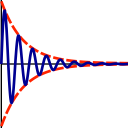SubsectionsSynopsis

Read R1, R2, NOE, or R2eff relaxation data from a file.

Defaults

relax_data.read(ri_id=None, ri_type=None, frq=None, file=None, dir=None, spin_id_col=None, mol_name_col=None, res_num_col=None, res_name_col=None, spin_num_col=None, spin_name_col=None, data_col=None, error_col=None, sep=None, spin_id=None)

Keyword arguments

ri_id: The relaxation data ID string. This must be a unique identifier.

ri_type: The relaxation data type, i.e. `R1', `R2', `NOE', or `R2eff'.

frq: The exact proton frequency of the spectrometer in Hertz. See the `sfrq' parameter in the Varian procpar file or the `SFO1' parameter in the Bruker acqus file.

file: The name of the file containing the relaxation data.

dir: The directory where the file is located.

spin_id_col: The spin ID string column (an alternative to the mol, res, and spin name and number columns).

mol_name_col: The molecule name column (alternative to the spin_id_col).

res_num_col: The residue number column (alternative to the spin_id_col).

res_name_col: The residue name column (alternative to the spin_id_col).

spin_num_col: The spin number column (alternative to the spin_id_col).

spin_name_col: The spin name column (alternative to the spin_id_col).

data_col: The relaxation data column.

error_col: The experimental error column.

sep: The column separator (the default is white space).

spin_id: The spin ID string to restrict the loading of data to certain spin subsets.

Description

This will load the relaxation data into the relax data store. The data is associated with the spectrometer frequency in Hertz. For subsequent analysis, this frequency must be set to the exact field strength. This value is stored in the `sfrq' parameter in the Varian procpar file or the `SFO1' parameter in the Bruker acqus file.

The spin system can be identified in the file using two different formats. The first is the spin ID string column which can include the molecule name, the residue name and number, and the spin name and number. Alternatively the molecule name, residue number, residue name, spin number and/or spin name columns can be supplied allowing this information to be in separate columns. Note that the numbering of columns starts at one. The spin ID string can be used to restrict the reading to certain spin types, for example only 15N spins when only residue information is in the file.

Prompt examples

The following commands will read the protein NOE relaxation data collected at 600 MHz out of a file called `noe.600.out' where the residue numbers, residue names, data, errors are in the first, second, third, and forth columns respectively.

[numbers=none]
relax> relax_data.read('NOE_600', 'NOE', 599.7 * 1e6, 'noe.600.out', res_num_col=1, res_name_col=2, data_col=3, error_col=4)

[numbers=none]
relax> relax_data.read(ri_id='NOE_600', ri_type='NOE', frq=600.0 * 1e6, file='noe.600.out', res_num_col=1, res_name_col=2, data_col=3, error_col=4)

The following commands will read the R2 data out of the file `r2.out' where the residue numbers, residue names, data, errors are in the second, third, fifth, and sixth columns respectively. The columns are separated by commas.

[numbers=none]
relax> relax_data.read('R2_800', 'R2', 8.0 * 1e8, 'r2.out', res_num_col=2, res_name_col=3, data_col=5, error_col=6, sep=',')

[numbers=none]
relax> relax_data.read(ri_id='R2_800', ri_type='R2', frq=8.0*1e8, file='r2.out', res_num_col=2, res_name_col=3, data_col=5, error_col=6, sep=',')

The following commands will read the R1 data out of the file `r1.out' where the columns are separated by the symbol `%'

[numbers=none]
relax> relax_data.read('R1_300', 'R1', 300.1 * 1e6, 'r1.out', sep='%')

The relax user manual (PDF), created 2020-08-26.